# Scattering by a Diffraction Grating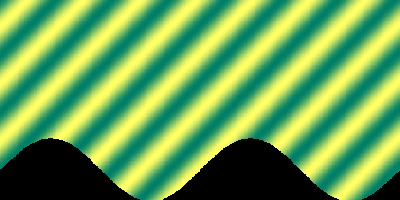A diffraction grating problem is the problem of scattering a plane incident wave by a periodic surface. We will here consider the case, when the total field vanishes on the surface. This corresponds, for example, to a TE polarised electromagnetic wave incident onto a perfectly conducting surface.

Mathematically, the problem is posed as a boundary value problem for the scattered field in the unbounded domain above the periodic surface, which is given as the graph o f a function f. The governing equation is the Helmholtz equation,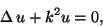and the boundary condition is that the sum of scattered and incident field vanishes on f.

To ensure uniqueness of solution to the mathematic boundary value problems, the scattered field will be assumed to be quasi-periodic, i.e. for some a > 0,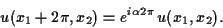and, as a radiation condition, will be assumed to have an expansion of the formfor some x2 > max f, where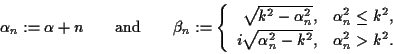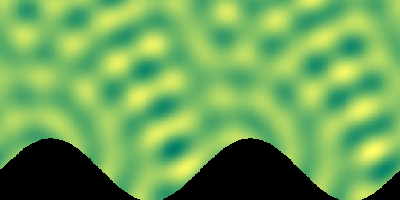A further consequence of these assumptions is that the problem can be formulated equivalently through a boundary integral equation that only involves an integral over a single period and thus is finite. Thus the corresponding integral operator can be shown to be compact.

The images on this page show the incident and scattered fields for such a problem involving a sinusoidal surface. The fields were computed by a numerical software package implemented by the Brunel University Rough Surface Scattering group, based on an exponentially convergent Nyström method for the solution of the boundary integral equation.

Below, you can view an animation of the total field for this problem using a Java applet. Click the button to download the animation data and start viewing.

Your browser is completely ignoring the <APPLET> tag!

Page written and maintained by Tilo Arens
last change: 24 July 2000
Mathematical formulae by latex2html
Java animation applet by Markus Kraft and Tilo Arens